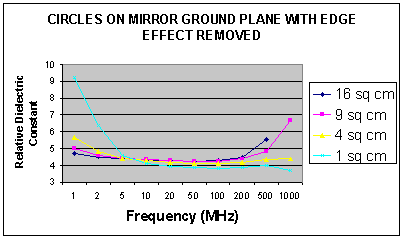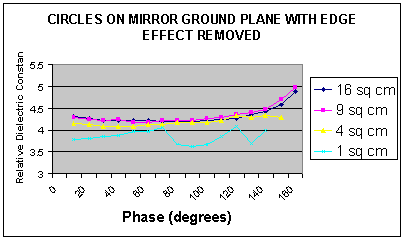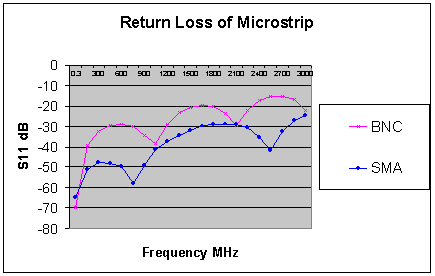# Measuring Relative Dielectric Constant of PCB's Using Parallel Plate Method and a Vector Network Analyser page 3

The εr as recalculated and the results are shown below in Figure 4.

Figure 4Figure 4 shows that the measured εr still varies with both frequency and sample size, but the curves of the two larger samples now coincide at 10 to 100 MHz. The curve of the smallest (1 cm2 ) sample has moved considerably from the other three and it is obvious that this sample is too small for accurate results. A closer examination shows that the 4 cm2 sample is also inadequate and it is not until the sample size reaches 16 cm2 that a reasonably smooth curve is produced. If the smallest sample is ignored, the minimum spread is now 1.3% at 5 MHz.

The next problem was to find the actual εr from the recalculated data. The text books tell us that the phase shift across a capacitor is 900, so the measurements were repeated at ten degree phase intervals. The results were again calculated with Formula 4 and are shown below in Figure 5.

Figure 5.If the smallest sample is again ignored, the minimum spread is now 0.85% at 900. We are now in the right ball park area for measurement accuracy and a check is warranted.

PCB-D

This has one circular sample 16 cm2 on the top, and a mirrored ground plane on the bottom. The VNA was calibrated and the phase length of the semi rigid cable was removed from the test by performing a port extension. The capacitance at 900 was measured at the capacitor centre and the εr calculated at 4.254 using the circular capacitor method.

PCB-E

This PCB was manufactured from the same sheet as PCB-D in order to obtain a similar εr. This has a calculated 50Ω microstrip transmission line and the PCB fits into a FLEXI-BOX for the measurement. The track width was calculated at 2.87 mm using the microstrip calculator. This calculator was later updated and now indicates 2.861 mm for a 50Ω microstrip. However, the results for the 2.87 mm track are excellent and are shown below in Figures 6&7.

Figure 61  2  3  4  5  >  Page

Technical Article Index